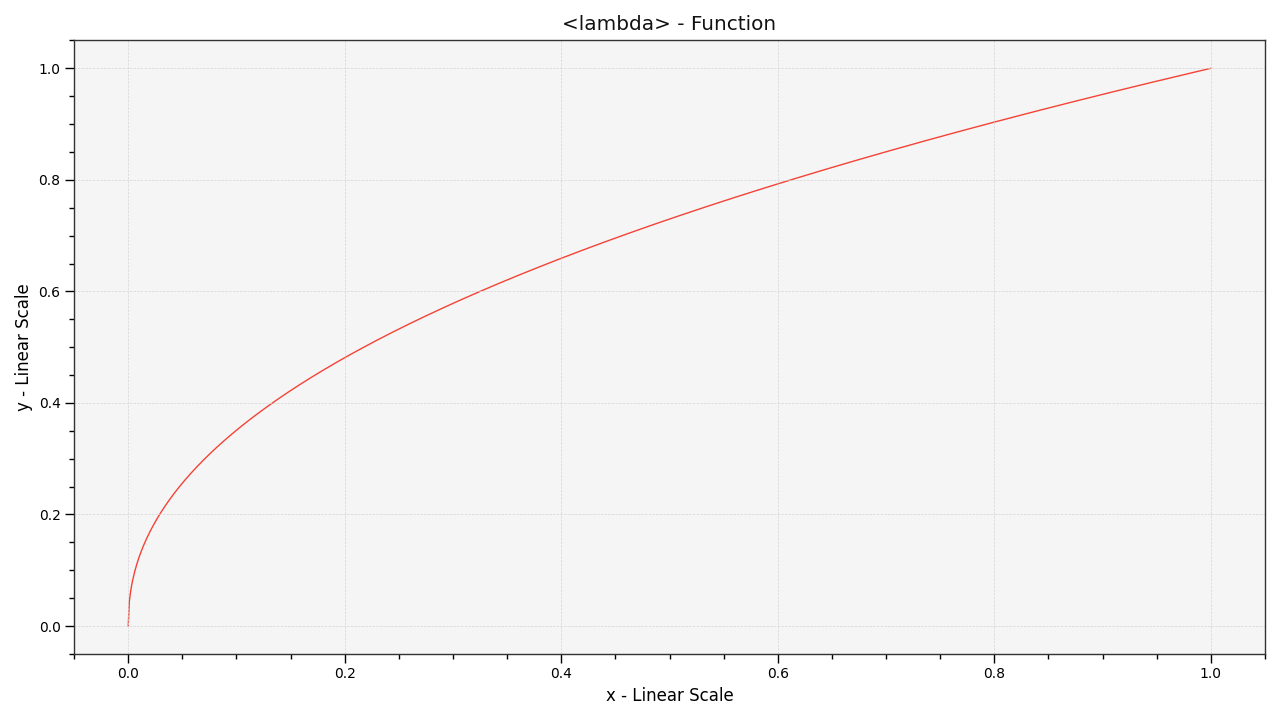# colour.plotting.plot_single_function¶

colour.plotting.plot_single_function(function, samples=None, log_x=None, log_y=None, **kwargs)[source]

Plots given function.

Parameters
• function (callable, optional) – Function to plot.

• samples (array_like, optional,) – Samples to evaluate the functions with.

• log_x (int, optional) – Log base to use for the x axis scale, if None, the x axis scale will be linear.

• log_y (int, optional) – Log base to use for the y axis scale, if None, the y axis scale will be linear.

Other Parameters

**kwargs (dict, optional) – {colour.plotting.artist(), colour.plotting.plot_multi_functions(), colour.plotting.render()}, Please refer to the documentation of the previously listed definitions.

Returns

Current figure and axes.

Return type

tuple

Examples

>>> from colour.models import gamma_function
>>> plot_single_function(partial(gamma_function, exponent=1 / 2.2))
...
(<Figure size ... with 1 Axes>, <matplotlib.axes._subplots.AxesSubplot object at 0x...>)